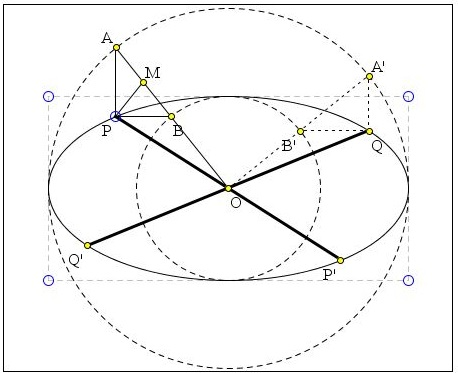## Between Major and Minor Circles

In a polar system of coordinates based at the center of ellipse, an ellipse is presented as a curve

P(t) = (a cos(t), b sin(t)),

where a and b are the lengths of its half-axes. Consider two circles (one major, the other minor) associated with the ellipse:

A(t) = (a cos(t), a sin(t)),
B(t) = (b cos(t), b sin(t)).

Both touch the ellipse at its vertices. If components of a generic point U = (p, q) are denoted p = Ux and q = Uy, we can say that

P(t) = (A(t)x, B(t)y).

This explains the configuration illustrated by the applet below.

Created with GeoGebra

Since ΔABP is right, the midpoint M of its hypotenuse AB is also the circumcenter: MA = MB = MP. Triangles AMP and BMP are isosceles. By the construction, if O is the center of the ellipse, OA = a, OB = b. It follows that

OM = (a + b) / 2.
MP = |a - b| / 2.

Let zX be the complex number associated with a point X. Then arg(zM) is the angle formed by the radius-vector OM with the (positive) x-axis. For a > b, the angle formed by MP with the (positive) x-axis is -arg(zM).If zA = aeit and zB = beit, zM = (a + b)/2·eit and

zP = (a + b)/2·eit + (a - b)/2·e-it.

(Euler's formula shows that this is exactly the same as zP = a cos(t) + ib sin(t), i.e., P = P(t) = (a cos(t), b sin(t)), as expected.)

In an ellipse, conjugate diameters correspond to complementary values of parameter t. The applet illustrates this point too: OA is perpendicular to OA', although PP' and QQ' are not perpendicular, except when they coincide with the axes of the ellipse.### Conic Sections > Ellipse